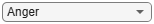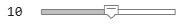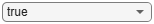# Speech Emotion Recognition

This example illustrates a simple speech emotion recognition (SER) system using a BiLSTM network. You begin by downloading the data set and then testing the trained network on individual files. The network was trained on a small German-language database .

The example walks you through training the network, which includes downloading, augmenting, and training the dataset. Finally, you perform leave-one-speaker-out (LOSO) 10-fold cross validation to evaluate the network architecture.

The features used in this example were chosen using sequential feature selection, similar to the method described in Sequential Feature Selection for Audio Features.

Download the Berlin Database of Emotional Speech . The database contains 535 utterances spoken by 10 actors intended to convey one of the following emotions: anger, boredom, disgust, anxiety/fear, happiness, sadness, or neutral. The emotions are text independent.

```dataFolder = tempdir; dataset = fullfile(dataFolder,"Emo-DB"); if ~datasetExists(dataset) url = "http://emodb.bilderbar.info/download/download.zip"; disp("Downloading Emo-DB (40.5 MB) ...") unzip(url,dataset) end```
```Downloading Emo-DB (40.5 MB) ... ```

Create an `audioDatastore` that points to the audio files.

`ads = audioDatastore(fullfile(dataset,"wav"));`

The file names are codes indicating the speaker ID, text spoken, emotion, and version. The website contains a key for interpreting the code and additional information about the speakers such as gender and age. Create a table with the variables `Speaker` and `Emotion`. Decode the file names into the table.

```filepaths = ads.Files; emotionCodes = cellfun(@(x)x(end-5),filepaths,UniformOutput=false); emotions = replace(emotionCodes,["W","L","E","A","F","T","N"], ... ["Anger","Boredom","Disgust","Anxiety/Fear","Happiness","Sadness","Neutral"]); speakerCodes = cellfun(@(x)x(end-10:end-9),filepaths,UniformOutput=false); labelTable = cell2table([speakerCodes,emotions],VariableNames=["Speaker","Emotion"]); labelTable.Emotion = categorical(labelTable.Emotion); labelTable.Speaker = categorical(labelTable.Speaker); summary(labelTable)```
```Variables: Speaker: 535×1 categorical Values: 03 49 08 58 09 43 10 38 11 55 12 35 13 61 14 69 15 56 16 71 Emotion: 535×1 categorical Values: Anger 127 Anxiety/Fear 69 Boredom 81 Disgust 46 Happiness 71 Neutral 79 Sadness 62 ```

`labelTable` is in the same order as the files in `audioDatastore`. Set the `Labels` property of the `audioDatastore` to the `labelTable`.

`ads.Labels = labelTable;`

### Perform Speech Emotion Recognition

Download and load the pretrained network, the `audioFeatureExtractor` object used to train the network, and normalization factors for the features. This network was trained using all speakers in the data set except speaker `03`.

```downloadFolder = matlab.internal.examples.downloadSupportFile("audio","SpeechEmotionRecognition.zip"); dataFolder = tempdir; unzip(downloadFolder,dataFolder) netFolder = fullfile(dataFolder,"SpeechEmotionRecognition"); load(fullfile(netFolder,"network_Audio_SER.mat"));```

The sample rate set on the `audioFeatureExtractor` corresponds to the sample rate of the data set.

`fs = afe.SampleRate;`

Select a speaker and emotion, then subset the datastore to only include the chosen speaker and emotion. Read from the datastore and listen to the file.

```speaker =categorical("03"); emotion =categorical("Disgust"); adsSubset = subset(ads,ads.Labels.Speaker==speaker & ads.Labels.Emotion==emotion); audio = read(adsSubset); sound(audio,fs)```

Use the `audioFeatureExtractor` object to extract the features and then transpose them so that time is along rows. Normalize the features and then convert them to 20-element sequences with 10-element overlap, which corresponds to approximately 600 ms windows with 300 ms overlap. Use the supporting function, HelperFeatureVector2Sequence, to convert the array of feature vectors to sequences.

```features = (extract(afe,audio))'; featuresNormalized = (features - normalizers.Mean)./normalizers.StandardDeviation; numOverlap =10; featureSequences = HelperFeatureVector2Sequence(featuresNormalized,20,numOverlap);```

Feed the feature sequences into the network for prediction. Compute the mean prediction and plot the probability distribution of the chosen emotions as a pie chart. You can try different speakers, emotions, sequence overlap, and prediction average to test the network's performance. To get a realistic approximation of the network's performance, use speaker `03`, which the network was not trained on.

```YPred = double(predict(net,featureSequences)); average ="mode"; switch average case "mean" probs = mean(YPred,1); case "median" probs = median(YPred,1); case "mode" probs = mode(YPred,1); end pie(probs./sum(probs),string(net.Layers(end).Classes))```The remainder of the example illustrates how the network was trained and validated.

### Train Network

The 10-fold cross validation accuracy of a first attempt at training was about 60% because of insufficient training data. A model trained on the insufficient data overfits some folds and underfits others. To improve overall fit, increase the size of the dataset using `audioDataAugmenter`. 50 augmentations per file was chosen empirically as a good tradeoff between processing time and accuracy improvement. You can decrease the number of augmentations to speed up the example.

Create an `audioDataAugmenter` object. Set the probability of applying pitch shifting to `0.5` and use the default range. Set the probability of applying time shifting to `1` and use a range of `[-0.3,0.3]` seconds. Set the probability of adding noise to `1` and specify the SNR range as `[-20,40]` dB.

```numAugmentations =50; augmenter = audioDataAugmenter(NumAugmentations=numAugmentations, ... TimeStretchProbability=0, ... VolumeControlProbability=0, ... ... PitchShiftProbability=0.5, ... ... TimeShiftProbability=1, ... TimeShiftRange=[-0.3,0.3], ... ... AddNoiseProbability=1, ... SNRRange=[-20,40]);```

Create a new folder in your current folder to hold the augmented data set.

```currentDir = pwd; writeDirectory = fullfile(currentDir,"augmentedData"); mkdir(writeDirectory)```

For each file in the audio datastore:

1. Create 50 augmentations.

2. Normalize the audio to have a max absolute value of 1.

3. Write the augmented audio data as a WAV file. Append `_``augK` to each of the file names, where K is the augmentation number. To speed up processing, use `parfor` and partition the datastore.

This method of augmenting the database is time consuming and space consuming. However, when iterating on choosing a network architecture or feature extraction pipeline, this upfront cost is generally advantageous.

```N = numel(ads.Files)*numAugmentations; reset(ads) numPartitions = 18; tic parfor ii = 1:numPartitions adsPart = partition(ads,numPartitions,ii); while hasdata(adsPart) [x,adsInfo] = read(adsPart); data = augment(augmenter,x,fs); [~,fn] = fileparts(adsInfo.FileName); for i = 1:size(data,1) augmentedAudio = data.Audio{i}; augmentedAudio = augmentedAudio/max(abs(augmentedAudio),[],"all"); augNum = num2str(i); if numel(augNum)==1 iString = ['0',augNum]; else iString = augNum; end audiowrite(fullfile(writeDirectory,sprintf('%s_aug%s.wav',fn,iString)),augmentedAudio,fs); end end end disp("Augmentation complete in " + round(toc/60,2) + " minutes.")```
```Augmentation complete in 3.84 minutes. ```

Create an audio datastore that points to the augmented data set. Replicate the rows of the label table of the original datastore `NumAugmentations` times to determine the labels of the augmented datastore.

```adsAug = audioDatastore(writeDirectory); adsAug.Labels = repelem(ads.Labels,augmenter.NumAugmentations,1);```

Create an `audioFeatureExtractor` object. Set `Window` to a periodic 30 ms Hamming window, `OverlapLength` to `0`, and `SampleRate` to the sample rate of the database. Set `gtcc`, `gtccDelta`, `mfccDelta`, and `spectralCrest` to `true` to extract them. Set `SpectralDescriptorInput` to `melSpectrum` so that the `spectralCrest` is calculated for the mel spectrum.

```win = hamming(round(0.03*fs),"periodic"); overlapLength = 0; afe = audioFeatureExtractor( ... Window=win, ... OverlapLength=overlapLength, ... SampleRate=fs, ... ... gtcc=true, ... gtccDelta=true, ... mfccDelta=true, ... ... SpectralDescriptorInput="melSpectrum", ... spectralCrest=true);```

### Train for Deployment

When you train for deployment, use all available speakers in the data set. Set the training datastore to the augmented datastore.

`adsTrain = adsAug;`

Convert the training audio datastore to a tall array. If you have Parallel Computing Toolbox™, the extraction is automatically parallelized. If you do not have Parallel Computing Toolbox™, the code continues to run.

`tallTrain = tall(adsTrain);`

Extract the training features and reorient the features so that time is along rows to be compatible with `sequenceInputLayer` (Deep Learning Toolbox).

```featuresTallTrain = cellfun(@(x)extract(afe,x),tallTrain,UniformOutput=false); featuresTallTrain = cellfun(@(x)x',featuresTallTrain,UniformOutput=false); featuresTrain = gather(featuresTallTrain);```
```Evaluating tall expression using the Parallel Pool 'local': - Pass 1 of 1: 0% complete Evaluation 0% complete - Pass 1 of 1: Completed in 1 min 7 sec Evaluation completed in 1 min 7 sec ```

Use the training set to determine the mean and standard deviation of each feature.

```allFeatures = cat(2,featuresTrain{:}); M = mean(allFeatures,2,"omitnan"); S = std(allFeatures,0,2,"omitnan"); featuresTrain = cellfun(@(x)(x-M)./S,featuresTrain,UniformOutput=false);```

Buffer the feature vectors into sequences so that each sequence consists of 20 feature vectors with overlaps of 10 feature vectors.

```featureVectorsPerSequence = 20; featureVectorOverlap = 10; [sequencesTrain,sequencePerFileTrain] = HelperFeatureVector2Sequence(featuresTrain,featureVectorsPerSequence,featureVectorOverlap);```

Replicate the labels of the training and validation sets so that they are in one-to-one correspondence with the sequences. Not all speakers have utterances for all emotions. Create an empty `categorical` array that contains all the emotional categories and append it to the validation labels so that the categorical array contains all emotions.

```labelsTrain = repelem(adsTrain.Labels.Emotion,[sequencePerFileTrain{:}]); emptyEmotions = ads.Labels.Emotion; emptyEmotions(:) = [];```

Define a BiLSTM network using `bilstmLayer` (Deep Learning Toolbox). Place a `dropoutLayer` (Deep Learning Toolbox) before and after the `bilstmLayer` to help prevent overfitting.

```dropoutProb1 = 0.3; numUnits = 200; dropoutProb2 = 0.6; layers = [ ... sequenceInputLayer(afe.FeatureVectorLength) dropoutLayer(dropoutProb1) bilstmLayer(numUnits,OutputMode="last") dropoutLayer(dropoutProb2) fullyConnectedLayer(numel(categories(emptyEmotions))) softmaxLayer classificationLayer];```

Define training options using `trainingOptions` (Deep Learning Toolbox).

```miniBatchSize = 512; initialLearnRate = 0.005; learnRateDropPeriod = 2; maxEpochs = 3; options = trainingOptions("adam", ... MiniBatchSize=miniBatchSize, ... InitialLearnRate=initialLearnRate, ... LearnRateDropPeriod=learnRateDropPeriod, ... LearnRateSchedule="piecewise", ... MaxEpochs=maxEpochs, ... Shuffle="every-epoch", ... Verbose=false, ... Plots="Training-Progress");```

Train the network using `trainNetwork` (Deep Learning Toolbox).

`net = trainNetwork(sequencesTrain,labelsTrain,layers,options);`To save the network, configured `audioFeatureExtractor`, and normalization factors, set `saveSERSystem` to `true`.

```saveSERSystem =false; if saveSERSystem normalizers.Mean = M; normalizers.StandardDeviation = S; save("network_Audio_SER.mat","net","afe","normalizers") end```

### Training for System Validation

To provide an accurate assessment of the model you created in this example, train and validate using leave-one-speaker-out (LOSO) k-fold cross validation. In this method, you train using $\mathit{k}-1$ speakers and then validate on the left-out speaker. You repeat this procedure k times. The final validation accuracy is the average of the k folds.

Create a variable that contains the speaker IDs. Determine the number of folds: 1 for each speaker. The database contains utterances from 10 unique speakers. Use `summary` to display the speaker IDs (left column) and the number of utterances they contribute to the database (right column).

```speaker = ads.Labels.Speaker; numFolds = numel(speaker); summary(speaker)```
``` 03 49 08 58 09 43 10 38 11 55 12 35 13 61 14 69 15 56 16 71 ```

The helper function HelperTrainAndValidateNetwork performs the steps outlined above for all 10 folds and returns the true and predicted labels for each fold. Call `HelperTrainAndValidateNetwork` with the `audioDatastore`, the augmented `audioDatastore`, and the `audioFeatureExtractor`.

`[labelsTrue,labelsPred] = HelperTrainAndValidateNetwork(ads,adsAug,afe);`

Print the accuracy per fold and plot the 10-fold confusion chart.

```for ii = 1:numel(labelsTrue) foldAcc = mean(labelsTrue{ii}==labelsPred{ii})*100; disp("Fold " + ii + ", Accuracy = " + round(foldAcc,2)) end```
```Fold 1, Accuracy = 65.31 Fold 2, Accuracy = 68.97 Fold 3, Accuracy = 79.07 Fold 4, Accuracy = 71.05 Fold 5, Accuracy = 72.73 Fold 6, Accuracy = 74.29 Fold 7, Accuracy = 67.21 Fold 8, Accuracy = 85.51 Fold 9, Accuracy = 71.43 Fold 10, Accuracy = 67.61 ```
```labelsTrueMat = cat(1,labelsTrue{:}); labelsPredMat = cat(1,labelsPred{:}); figure cm = confusionchart(labelsTrueMat,labelsPredMat, ... Title=["Confusion Matrix for 10-Fold Cross-Validation","Average Accuracy = " round(mean(labelsTrueMat==labelsPredMat)*100,1)], ... ColumnSummary="column-normalized",RowSummary="row-normalized"); sortClasses(cm,categories(emptyEmotions))```### Supporting Functions

#### Convert Array of Feature Vectors to Sequences

```function [sequences,sequencePerFile] = HelperFeatureVector2Sequence(features,featureVectorsPerSequence,featureVectorOverlap) % Copyright 2019 MathWorks, Inc. if featureVectorsPerSequence <= featureVectorOverlap error("The number of overlapping feature vectors must be less than the number of feature vectors per sequence.") end if ~iscell(features) features = {features}; end hopLength = featureVectorsPerSequence - featureVectorOverlap; idx1 = 1; sequences = {}; sequencePerFile = cell(numel(features),1); for ii = 1:numel(features) sequencePerFile{ii} = floor((size(features{ii},2) - featureVectorsPerSequence)/hopLength) + 1; idx2 = 1; for j = 1:sequencePerFile{ii} sequences{idx1,1} = features{ii}(:,idx2:idx2 + featureVectorsPerSequence - 1); %#ok<AGROW> idx1 = idx1 + 1; idx2 = idx2 + hopLength; end end end```

#### Train and Validate Network

```function [trueLabelsCrossFold,predictedLabelsCrossFold] = HelperTrainAndValidateNetwork(varargin) % Copyright 2019 The MathWorks, Inc. if nargin == 3 ads = varargin{1}; augads = varargin{2}; extractor = varargin{3}; elseif nargin == 2 ads = varargin{1}; augads = varargin{1}; extractor = varargin{2}; end speaker = categories(ads.Labels.Speaker); numFolds = numel(speaker); emptyEmotions = (ads.Labels.Emotion); emptyEmotions(:) = []; % Loop over each fold. trueLabelsCrossFold = {}; predictedLabelsCrossFold = {}; for i = 1:numFolds % 1. Divide the audio datastore into training and validation sets. % Convert the data to tall arrays. idxTrain = augads.Labels.Speaker~=speaker(i); augadsTrain = subset(augads,idxTrain); augadsTrain.Labels = augadsTrain.Labels.Emotion; tallTrain = tall(augadsTrain); idxValidation = ads.Labels.Speaker==speaker(i); adsValidation = subset(ads,idxValidation); adsValidation.Labels = adsValidation.Labels.Emotion; tallValidation = tall(adsValidation); % 2. Extract features from the training set. Reorient the features % so that time is along rows to be compatible with % sequenceInputLayer. tallTrain = cellfun(@(x)x/max(abs(x),[],"all"),tallTrain,UniformOutput=false); tallFeaturesTrain = cellfun(@(x)extract(extractor,x),tallTrain,UniformOutput=false); tallFeaturesTrain = cellfun(@(x)x',tallFeaturesTrain,UniformOutput=false); %#ok<NASGU> [~,featuresTrain] = evalc('gather(tallFeaturesTrain)'); % Use evalc to suppress command-line output. tallValidation = cellfun(@(x)x/max(abs(x),[],"all"),tallValidation,UniformOutput=false); tallFeaturesValidation = cellfun(@(x)extract(extractor,x),tallValidation,"UniformOutput",false); tallFeaturesValidation = cellfun(@(x)x',tallFeaturesValidation,UniformOutput=false); %#ok<NASGU> [~,featuresValidation] = evalc('gather(tallFeaturesValidation)'); % Use evalc to suppress command-line output. % 3. Use the training set to determine the mean and standard % deviation of each feature. Normalize the training and validation % sets. allFeatures = cat(2,featuresTrain{:}); M = mean(allFeatures,2,"omitnan"); S = std(allFeatures,0,2,"omitnan"); featuresTrain = cellfun(@(x)(x-M)./S,featuresTrain,UniformOutput=false); for ii = 1:numel(featuresTrain) idx = find(isnan(featuresTrain{ii})); if ~isempty(idx) featuresTrain{ii}(idx) = 0; end end featuresValidation = cellfun(@(x)(x-M)./S,featuresValidation,UniformOutput=false); for ii = 1:numel(featuresValidation) idx = find(isnan(featuresValidation{ii})); if ~isempty(idx) featuresValidation{ii}(idx) = 0; end end % 4. Buffer the sequences so that each sequence consists of twenty % feature vectors with overlaps of 10 feature vectors. featureVectorsPerSequence = 20; featureVectorOverlap = 10; [sequencesTrain,sequencePerFileTrain] = HelperFeatureVector2Sequence(featuresTrain,featureVectorsPerSequence,featureVectorOverlap); [sequencesValidation,sequencePerFileValidation] = HelperFeatureVector2Sequence(featuresValidation,featureVectorsPerSequence,featureVectorOverlap); % 5. Replicate the labels of the train and validation sets so that % they are in one-to-one correspondence with the sequences. labelsTrain = [emptyEmotions;augadsTrain.Labels]; labelsTrain = labelsTrain(:); labelsTrain = repelem(labelsTrain,[sequencePerFileTrain{:}]); % 6. Define a BiLSTM network. dropoutProb1 = 0.3; numUnits = 200; dropoutProb2 = 0.6; layers = [ ... sequenceInputLayer(size(sequencesTrain{1},1)) dropoutLayer(dropoutProb1) bilstmLayer(numUnits,OutputMode="last") dropoutLayer(dropoutProb2) fullyConnectedLayer(numel(categories(emptyEmotions))) softmaxLayer classificationLayer]; % 7. Define training options. miniBatchSize = 512; initialLearnRate = 0.005; learnRateDropPeriod = 2; maxEpochs = 3; options = trainingOptions("adam", ... MiniBatchSize=miniBatchSize, ... InitialLearnRate=initialLearnRate, ... LearnRateDropPeriod=learnRateDropPeriod, ... LearnRateSchedule="piecewise", ... MaxEpochs=maxEpochs, ... Shuffle="every-epoch", ... Verbose=false); % 8. Train the network. net = trainNetwork(sequencesTrain,labelsTrain,layers,options); % 9. Evaluate the network. Call classify to get the predicted labels % for each sequence. Get the mode of the predicted labels of each % sequence to get the predicted labels of each file. predictedLabelsPerSequence = classify(net,sequencesValidation); trueLabels = categorical(adsValidation.Labels); predictedLabels = trueLabels; idx1 = 1; for ii = 1:numel(trueLabels) predictedLabels(ii,:) = mode(predictedLabelsPerSequence(idx1:idx1 + sequencePerFileValidation{ii} - 1,:),1); idx1 = idx1 + sequencePerFileValidation{ii}; end trueLabelsCrossFold{i} = trueLabels; %#ok<AGROW> predictedLabelsCrossFold{i} = predictedLabels; %#ok<AGROW> end end```

### References

 Burkhardt, F., A. Paeschke, M. Rolfes, W.F. Sendlmeier, and B. Weiss, "A Database of German Emotional Speech." In Proceedings Interspeech 2005. Lisbon, Portugal: International Speech Communication Association, 2005.